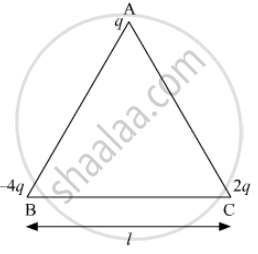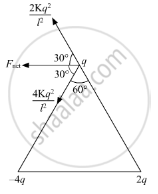# Three-point Charges Q, – 4q and 2q Are Placed at the Vertices of an Equilateral Triangle Abc of Side 'L' as Shown in the Figure. Obtain the Expression for the Magnitude of the Resultant Electric - Physics

Three-point charges q, – 4q and 2q are placed at the vertices of an equilateral triangle ABC of side 'l' as shown in the figure. Obtain the expression for the magnitude of the resultant electric force acting on the charge q(b) Find out the amount of the work done to separate the charges at infinite distance.

#### Solution$F_{net} = \frac{2K q^2}{l^2}\cos {30}^0 + \frac{4K q^2}{l^2}\cos {30}^0$
$= \frac{3\sqrt{3}K q^2}{l^2}$

(b) PEinitial

$- \frac{8K q^2}{l} - \frac{4K q^2}{l} + \frac{2K q^2}{l}$
$= - \frac{10K q^2}{l}$

PEfinal = 0

Workdone = PEfinal - PEinitial =

$\frac{10K q^2}{l}$
Concept: Coulomb’s Law - Force Between Two Point Charges
Is there an error in this question or solution?